×#### Thank you for registering.

One of our academic counsellors will contact you within 1 working day.

Click to Chat

1800-1023-196

+91-120-4616500

CART 0

• 0

MY CART (5)

Use Coupon: CART20 and get 20% off on all online Study Material

ITEM
DETAILS
MRP
DISCOUNT
FINAL PRICE
Total Price: Rs.

There are no items in this cart.
Continue Shopping```Objective Problems on Particular Integral

1. A curve that passes through (2, 4) and having subnormal of constant length of 8 units can be;

(A) y2 = 16x – 8                                                      (B) y2 = -16x + 24

(C) x2 = 16y – 60                                                    (D) x2 = -16y + 68

Solution:  Let the curve be y = f(x). Subnormal at any point = | y dy/dx|

So differentiating with respect to x we get,

y dy/dx = ±8 ⇒ y dy = ±8 dx ⇒ y 2/2 =   ±8x + c

y2 = 16 x+2c1, if c1= -8    or

y2 = -16x +2c2, and c2= 24

Hence  (A), (B) are  correct answers.

2. Equation of a curve that would cut x2 + y2 - 2x - 4y - 15 = 0 orthogonally can be;

(A) (y-2) = l(x-1)                                                 (B) (y-1) = l(x-2)

(C) (y+2) = l(x+1)                                                   (D) (y+1) = l(x+2)   where l ∈R.

Solution: Any line passing through the center of the given circle would meet the circle orthogonally.

Hence (A) is the correct answer.

3. Let m and n be the order and the degree of the differential equation whose solution is y = cx +c2- 3c3/2 +2, where c is a parameter. Then,

(A) m = 1, n = 4                                                               (B) m =1 , n =3

(C) m = 1, n = 2                                                          (D) None

Solution: Differentiating the solution we get, dy/dx = c.

Hence, the differential equation is given by y = x dy/dx + (dy/dx)2- 3 (dy/dx)3/2 +2.

Clearly its order is one and degree 4.

Hence (A) is the  correct answer.

4.   y = a sinx + b cosx  is the solution of differential  equation :

(A) d2y/dx2 + y = 0                                       (B) dy/dx + y = 0

(C) d2y/dx2 = y                                            (D) dy/dx = y

Solution:  With the help of the given y, we find dy/dx and d2y/dx2 and try to find the appropriate relation

dy/dx = a cos x –b sin x and d2y/dx2 = -a sinx –b cosx = -y

Hence d2y/dx2 +y =0.

Hence (A) is the correct answer.

5. For any differential function y = f(x), the value of d2y/dx2 + (dy/dx)3 d2y/dx2 is :

(A) Always zero                                              (B) always non-zero

(C) equal to 2y2                                              (D) equal to x2

Solution: It is obvious that (dy/dx) = (dx/dy)-1 for a differential equation

d2y/dx2 =-1 (dx/dy)-2 d/dy (dx/dy) dy/dx

= - (dx/dy)-2 d2x/dy2 dy/dx

= -d2x/dy2 (dy/dx)3

Hence, d2y/dx2 + (dy/dx)3 d2x/dy2 =0.

Hence (A) is the correct answer.

6. The degree of differential equation d2y/dx2 +3(dy/dx)2 = x log d2y/dx2  is :

(A) 1                                                                (B) 2

(C) 3                                                                 (D) none of these

Solution: Since the equation is not a polynomial in all the differential coefficients so the degree of the equation is not defined.

Hence (D) is the correct answer.

7. The degree and order of the differential equation of all parabolas whose axis is x-axis are:

(A) 2, 1                                                               (B) 1, 2

(C) 3, 2                                                               (D) none of these

Solution: Equation of required parabola is of the form y2 = 4a(x –h)

Differentiating, we have 2y dy/dx = 4a and so, y dy/dx = 2a

Required differential equation is (dy/dx)2 + d2y/dx2 y =0.

Degree of the equation is 1 and order is 2.

Hence (B) is the correct answer.

8. The differential equation of all ellipses centered at origin is:

(A) y2 + xy12 –yy1 = 0                                                 (B) xyy2 + xy12 –yy1 = 0

(C) yy2 + xy12 –xy1 = 0                                               (D) none of these

Solution: Ellipse centered at origin are given by

x2/a2 + y2/b2 =1                    …… (1)

where a and b are unknown constants

2x/a2 +2y/b2 y1 =0, where y1 = dy/dx

x/a2 +y/b2 .y1 = 0                   …… (2)

Differentiating again we get, 1/a2 +1/b2 (y12 +yy2) =0            ……. (3)

Multiplying (3) with x and then subtracting from (2) we get

1/b2 (yy1 – xy12 –xyy2) = 0 so  xyy2 + xy12 –yy1 = 0.

Hence (B) is the correct answer.

9. Particular solution of y1 + 3xy = x which passes through (0, 4) is :

(A) 3y = 1 + 11(B) y =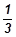+ 11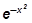(C) y = 1 +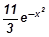(D) y =+ 11Solution: dy/dx + (3x) y = x

I.F =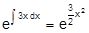Solution of given equation is

y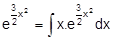+ c =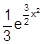+ c

If curve passes through (0, 4), then

4 –1/3 = c and c = 11/3

y =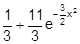so 3y = 1 + 11.

Hence (A) is the correct answer.

10. Solution of equation dy/dx = (3x-4y-2)/(3x-4y-3) is :

(A) (x –y)2 + c = log (3x –4y + 1)                    (B) x –y + c = log (3x –4y + 1)

(C) x –y + c = = log (3x –4y –3)                      (D) x –y + c = log (3x –4y + 1)

Solution: Let 3x –4y = z

3-4dy/dx = dz/dx and dy/dx = ¼ (3-dz/dx)

Therefore the given equation ¾ - ¼ dz/dx = (z-2)/ (z-3)

Hence, (z-3) / (-z-1) dz = dx.

Solving we get, –z + 4 log (z + 1) = x + c

This gives log (3x –4y + 1) = x –y + c.

Hence (B) is the correct answer.

11. The order of the differential equation, whose general solution is

y = C1 ex + C2 e2x + C3 e3x + C4 ex+c3, where C1, C2, C3, C4, C5 are arbitrary constants, is:

(A) 5                                                          (B) 4

(C) 3                                                          (D) none of these

Solution: y = (c1 + c4) ex + c2 e2x + c3 e3x + c4ec5

y = c1 ex + c2 e2x + c3 e3x + c4ec5

y = k1 ex + k2 e2x + k3 e3x + k­4

Therefore there are 4 arbitrary constants

Hence (B) is the correct answer.

12. I.F. for y ln y dx/dy + x – ln y =0 is:

(A) ln x                                      (B) ln y

(C) ln xy                                      (D) none of these

Solution:          I.F. = e ∫dy/dx = ln y

Hence (B) is the correct answer.

13. Which one of the following is a differential equation of the family of curves

y =Ae2x + Be-2x

(A) d2y/dx2 -2 dy/dx +2y =0                 (B) xd2y/dx2 +2dy/dx –xy + x2 -2 = 0

(B)  d2y/dx2 = 4y                                   (D) (dy/dx)3 = 4y (x dy/dx -2y)

Solution: Given equation is y = A e2x + b e-2x

So dy/dx = 2 (A e2x- b e-2x)

d2y/dx2 = 4 (Ae2x + be-2x) = ln y

Hence (C) is the correct answer.

14. Solution of dy/dx + sin [(x+y)/2] = sin(x-y/2) is:

(A) log tan y/4 = c – 2 sin x/2                           (B) log cot y/4 = c – 2 sin x/2

(C) log tan y/4 = c – 2 cos x/2                           (D) none of these

Solution:     dy/dx = - 2 cos x/2 sin y/2

∫ 2 cos x/2 dx = ∫ cosec y/2 dy and so c-2 sin x/2 = log tan y/4.

Hence (A) is the correct answer.

15. Solution of dy/dx = y/x + tan y/x is:

(A) sin (y/x) = kx                                          (B) cos (y/x)  = kx

(C) tan (y/x)  = kx                                          (D) none of these

Solution: dy/dx = y/x + tan y/x

put y = vx then v + x dv/dx = v + tan v

cot v dv = dx/x

Integrating, we get ln sin v = ln x + ln k

Hence, sin y/x = kx

Hence (A) is the correct answer.

16. Solution of dy/dx + √ (1-y2) / (1-x2) =0 is:

(A) sin–1 x – sin–1 y = c                            (B) sin–1 y + sin–1 x = c

(C) sin–1 x = c sin–1 y                                (D) (sin–1 x) (sin–1 y) = c

Solution:  dy/ √ (1-y2) = -dx /√ (1-x2)

So sin-1 y + sin-1 x = c

Hence (B) is the correct answer.

17. General solution of d2y/dx2 = e–2x is:

(A) y = ¼ e–2x + c                                            (B) y = e–2x + cx + d

(C) y = ¼ e–2x + cx + d                                    (D) y = e–2x + cx2 + d

Solution:    d2y/dx2 = e–2x

Hence, by integrating we get dy/dx = e–2x/2+k1

Integrating, y = e–2x/4 + k1x + k2  and then y = e–2x/4 + cx + d

Hence (C) is the correct answer.

18. Solution of dy/dx +y/x = x2 is:

(A) x + y = x2/2 + c                                        (B) x  – y = x3/3 + c

(C) xy = x4 + c                                                (D) y – x = x4 + c

Solution:   dy/dx +y/x = x2

I.F. = e ∫ dx/x = x

Therefore solution is xy = ∫x2. x dx= x4 / 4 +c.

Hence (C) is the correct answer.

19. Differential equation associated with primitive y = Ae3x + Be5x :

(A) d2y/dx2 -8 dy/dx +15y = 0.          (B)   d2y/dx2 + 8 dy/dx -15y =0

(C) d2y/dx2 + 8 dy/dx + y =0             (D) none of these

Solution: y = A e3x + B e5x

y1 = 3 A e3x + 5 B e5x

y2= 9 A e3x + 25 B e5x

Therefore y2- 8y + 15y = 0

Hence (A) is the correct answer.

20. The curve satisfying y = 2x dy/dx is a:

(A)  Family of parabola                            (B) family of circles

(C) Pair of straight line                           (D) none of these

Solution:   dx/dy = 2dy/y

Hence, ln y = ln y2 + ln c

Then y2 = kx. It clearly represents a family of parabola

Hence (A) is the correct answer.

21. The equation of the curve through the origin satisfying the equation dy = (sec x + y tanx) dx is:

(A) y sin =x                                                (B) y cosx = x

(C) y tanx = x                                                 (D) none of these

Solution: dx/dy - y tan x = sec x

I.F. = e - ∫ tan x dx = cos x

y cos x = ∫ sec x cos x dx + c

y cos x = x + c

At (0, q), y cos x = x          since c = 0

Hence (B) is the correct answer.

22. The differential equation y dy/dx + x = a (where ‘a’ is a constant) represents:

(A) A set of circles having center on y-axis

(B) A set of circles with center on x-axis

(C) A set of ellipses

(D) none of these

Solution: y dy = (a - x) dx

y2/ 2 = ax –x2/2

x2 + y2 = 2ax

Hence, x2 + 2ax + y2 = 0

It represents a set of sides with center on x-axis

Hence (B) is the correct answer.

23. If dy/dx = e–2y and y = 0 when x = 5, then value of x where y = 3 is given by:

(A) e5                                                          (B) (e6 +9) / 2

(C) e6 + 1                                                     (D) loge 6

Solution:  e2y dy = dx

Hence, e2y /2 + c = x

Then, c = 5 – ½ = 9/2.

At y = 3, the value of x is (e6 + 9) / 2

Hence (B) is the correct answer.

24. The equation of curve passing through (2,7/2) and having slope 1- 1/x2 at (x, y) is:

(A) y = x2 + x + 1                                             (B) xy = x + 1

(C) xy = x2 + x + 1                                           (D) xy = y + 1

Solution:  Since the slope is given, we have dy/dx = 1- 1/x2

y = x + 1/x + c

At (2, 7/2) the value becomes 7/2 = 2 + 1/2+ c

Hence, this gives c=1.

Therefore y = x + 1/x + 1

xy = x2 + x + 1

Hence (C) is the correct answer.

25. Given that dy/dx = yex such that x = 0, y = e. The value of y (y > 0) when x = 1 will be:

(A) e                                                     (B) 1/e

(C) e                                                      (D) e2

Solution: We are given that dy/dx = yex

Hence, dy/y = ex dx

Integrating both sides we get, ln y = ex + c

At x = 0, y = 1; c = 0

This gives, ln y = ex

Therefore At x = 1, y = ec

Hence (C) is the correct answer.

26. The differential equation of all conics with center at origin is of order

(A) 2                                               (B) 3

(C) 4                                               (D) None of these

Solution: The general equation of all conics with center at origin is

ax2 + 2hxy + by2 = 1

Since it has three arbitrary constants, the differential equation is of order 3.

Hence (B) is the correct answer.

27. The differential equation of all conics with the coordinate axes, is of order

(A)  1                                             (B) 2

(C)  3                                             (D) 4

Solution: The general equation of all conics with axes as coordinate axes is

ax2 + by2 = 1.

Since it has two arbitrary constants, the differential equation is of order 2.

Hence (B) is the correct answer.

28. The differential equation of all non-vertical lines in a plane is:

(A) dy/dx = 0                               (B) dx/dy =0

(C) d2y/dx2=0                            (D) d2y/dx2=0

Solution: The general equation of non-vertical lines in a plane is

ax + by = 1, b ≠ 0

Then a+bdy/dx = 0 ⇒ dy/dx = -a/b

Integrating it again, we get d2y/dx2 = 0.

Hence (C) is the correct answer.

29. The differential equation of all non-horizontal lines in a plane is:

(A) dy/dx = 0                                             (B) dx/dy =0

(C) d2y/dx2 =0                                            (D) d2x/dy2 =0

Solution: We know that the equation of non- horizontal ax = by = 0, a ≠ 0

Hence, the required differential equation will be given by d2x/dy2 =0.

Hence (D) is the correct answer.

30. The degree and order of the differential equation of all tangent lines to the parabola x2 = 4y is:

(A) 2, 1                                           (B) 2, 2

(C) 1, 3                                       (D) 1, 4

Solution: Equation of any tangent to x2 = 4y is

x = my+1/m, where m is arbitrary constant …… (1)

Now differentiating this equation we get,

1= m dy/dx

⇒ m = 1/ (dy/dx)

Now putting this value of m in equation (1) we get

x = y. 1/(dy/dx) + dy/dx

Solving it we obtain the equation

(dy/dx)2 –xdy/dx + y = 0.

which is clearly a differential equation of order 1 and degree 2.

Hence (A) is the correct answer.

31. If f(x) = f'(x) and f(1) = 2, then f(3) =

(A) e2                                       (B) 2e2

(C) 3e2                                      (D) 2e3

Solution: Since f(x) = f'(x)

⇒ f '(x)/f(x) = 1

⇒ Integrating we get log f(x) = x + c

Since f (1) = 2

This gives log 2 = 1 + c

log f(x) = x + log 2 – 1

log f(3) = 3 + log 2 – 1

= 2 + log 2

⇒ f(3) = e2+log2 = e2 . elog2 = 2e2.

Hence (B) is the correct answer.

32. Equation of the curve passing through (3, 9) which satisfies the differential equation dy/dx = x+1/x2 is:

(A)    6xy = 3x2 – 6x + 29                      (B)         6xy = 3x2 – 29x + 6

(C)     6xy = 3x3 – 29x – 6                      (D)        None of these

Solution: The given differential equation is

dy/dx = x +1/x2

Integrating both sides we get, y= x2/2 -1/x + c

It passes through (3, 9)

Substituting these values in the last equation we get,

9= 9/2-1/3 +c ⇒ c=29/6.

y= x2/2 -1/x +29/6

Hence (C) is the correct answer.

33. Solution of 2y sinx dy/dx = 2sinx cosx –y2cosx, x=p/2, y=1 is given by

(A) y2 = sinx                                   (B) y = sin2x

(C) y2 = cosx + 1                             (D) None of these

Solution: On dividing the given equation by sin x,

2ydy/dx +y2cot x = 2cos x

Now put y2 =v, then 2y dy/dx = dv/dx

Then the given equation becomes

dv/dx + v cot x = 2 cos x

I.F. = e ∫ cot x dx = elog sinx = sin x

Solution is v. sin x = ∫ sinx .(2 cos x) dx + c

⇒ y2 sin x = sin2x + c

When x= p/2, y = 1, then c = 0

⇒ y2 = sin x.

Hence (A) is the correct answer.

34. The general solution of the equation dy/dx = 1+xy is:

(A)(B)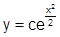(C)(D) None of these

Solution: Given equation is dy/dx = 1+xy

⇒ dy/dx –xy =1

This is the standeard form of linear equation and here, P=-x and Q=1

I.F. =Solution is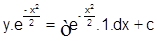Hence (D) is the correct answer.

35. Solution of dy/dx = e y+x + e y-x is:

(A) ex (x + 1) = y                            (B) ex (x + 1) + 1 = y

(C) ex (x – 1) + 1 = y                      (D) None of these

Solution: The given equation is dy/dx = e y+x + e y-x = ey.ex + ey.e-x = ey (ex+e-x)

e-y dy = (ex +e-x)dx

⇒ e–y dy = (ex + e–x)dx

Integrating we get, e–y = ex – e–x + c

Hence (D) is the correct answer.

36. If y = e–x (A cosx + B sin x), then y satisfies

(A) d2y/dx2 + 2dy/dx =0 =0                (B) d2y/dx2 - 2dy/dx +2y =0

(C) d2y/dx2 + 2dy/dx +2y =0               (D) d2y/dx2 + 2y =0

Solution: y = e–x (A cos x + B sin x)                            ....(1)

⇒ dy/dx = e-x (-A sin x +B cos x) - e-x (A cos x +B sin x)

⇒ dy/dx = e-x (-A sinx +B cos x) –y                              ……(2)

⇒ d2y/dx2 = e-x (-A cosx -B sin x) –e-x (-A sinx +B cos x)

Using (1) and (2), we get

d2y/dx2 +dy/dx = -y-y-dy/dx

d2y/dx2 +2dy/dx + 2y= 0.

Hence (C) is the correct answer.

37. The solution of x2 dy/dx –xy = 1+ cos y/x is:

(A) tan y/2x = c-1/2x2                   (B) tan y/x = c+1/x

(C) cos y/x =1+c/x                        (D) x2 =(c+x2) tan y/x

Solution: Dividing the given equation by x2 throughout

dy/dx –y/x = 1/x2 + 1/x2 cos y/x                          .....(1)

Put y = vx, then dy/dx = v +x dv/dx

Then equation (1) becomes v + x dv/dx –v =1/x2 +1/x2 cos v

⇒ x3 dv/dx = 1+cos v

Then dv/ (1+cos v) = dx/x3

Integrating both sides, ∫dv/ (1+cos v) = ∫dx/x3

½ sec2v/2 dv = -1/x +c

⇒ tan v/2 = -1/2x2 +c

Hence (A) is the correct answer.

38. The order of differential equation d2y/dx2 = 1+dy/dx is:

(A) 2                                   (B 3

(C) 1/2                                (D) 6

Solution: Clearly the equation is of order 2.

Hence (A) is the correct answer.

39. The solution of the differential equation 2x dy/dx –y =3 represents

(A) straight lines                       (B) circles

(C) parabolas                            (D) ellipses

Solution: The given equation is 2x dy/dx =y+3.

Hence, Separating the terms of x and y, we get

⇒ 2dy/(y+3) = dx/x

Integrating, 2log (y+3) = log x +log c

⇒ (y + 3)2 = ex, which represents a parabola.

Hence (C) is the correct answer.

40. Solution of differential equation dy – sinx siny dx = 0 is:

(A) e cosx tan y/2 = c                          (B) e cosx tan y = c

(C) cos x tan y = c                            (D) cos x sin y = c

Solution: Given equation can be written as:

dy/ siny = sinx dx

Now integrating both sides we get,

∫ cosec y dy = ∫ sinx dx +c

log tan y/2 = -cos x+c1

tan y/2 = e-cos x ec1

⇒ e cosx tan y/2 = c

Hence (A) is the correct answer.

41. Solution of differential equation dy/dx + ay =emx is:

(A) (a + m) y = emx + c                           (B) yeax = memx + c

(C) y = emx + ce–ax                                   (D) (a + m) y = emx + ce–ax

Solution: The given equation is dy/dx + ay =emx

This linear equation is already in the standard form and so P= a, Q= emx

I.F. = e ∫ adx = eax

Hence, the solution is y.eax = ∫ eax. emx dx +c

⇒ y eax =e(a+m)x / (a+m) +c1

⇒ (a + m) y = emx + c1 (a + m) e–ax

⇒ (a + m) y = emx + ce–ax, where c = c1 (a + m)

Hence (D) is the correct answer.

42. Integrating factor of the differential equation cos x dy/dx +y sin x=1 is:

(A) cos x                                  (B) tan x

(C) sec x                                   (D) sin x

Solution: The given equation is cos x dy/dx + y sin x=1

This can be rewritten as dy/dx + y sin x /cos x = 1/cos x

Here P= tan x, Q= sec x

I.F. = e ∫ tan x dx = elog secx = sec x.

Hence (C) is the correct answer.

43. Solution of differential equation xdy – ydx = 0 represents

(A) A rectangular hyperbola

(B) Straight line passing through origin

(C) Parabola whose vertex is at origin

(D) Circle whose center is at origin

Solution: Given equation is x dy – y dx = 0

⇒ dy/y –dx/x =0

⇒ log y – log x = log c

⇒ log y/x = log c

⇒ y/x =c or y=cx which is the equation of a straight line.

Hence (B) is the correct answer.

Watch this Video for more reference

44. The integrating factor of the differential equation

dy/dx (x log x) + y =2log x is given by

(A) ex                              (B) log x

(C) log (log x)                 (D) x

Solution: The given equation is

dy/dx (x log x) + y =2log x

Dividing throughout by xlog x the equation reduces in the form,

dy/dx + y/xlog x = 2/x

⇒ P =1/xlog x, Q = 2/x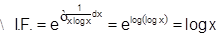Hence (B) is the correct answer.

45. The solution of dy/dx +1 = e x+y is:

(A) (x + y) ex+y = 0                    (B) (x + c) ex+y = 0

(C) (x – y) ex+y = 1                    (D) (x – c) ex+y + 1 = 0

Solution: The given equation is dy/dx + 1= ex+y

⇒ dy/dx +1 = ex.ey

⇒ e-y dy/dx +e-y = ex

Put e–y = z

⇒ -e–y dy/dx = dz/dx

Hence, the given equation becomes dz/dx - z = -ex

This is clearly a linear equation where P= -1 and Q= -ex

I.F. = e ∫–1dx = e–x

Solution is z  e–x =  ∫ –ex . e–x dx + c = –x + c

⇒ e–y .e–x = c – x

⇒ e–(x+y) = c – x

⇒ (x – c) ex+y + 1 = 0

Hence (D) is the correct answer.

46. Family y = Ax + A3 of curves is represented by the differential equation of degree

(A) 3                                          (B) 2

(C) 1                                          (D) None of these

Solution: There is only one arbitrary constant iinvolved and hence the degree of the differential equation is one.

Hence (C) is the correct answer.

47. Integrating factor of dy/dx +y/x = x3; (x > 0) is :

(A) x                                               (B) log x

(C) –x                                             (D) ex

Solution: I.F. is given by exp( ∫1/x dx) = x.

Hence (A) is the correct answer.

48. If integrating factor of x(1 – x2) dy + (2x2y – y – ax3) dx = 0 is e ∫ Pdx, then P is:

(A) (2x2 –ax3) / (x (1-x2))                     (B) 2x3 – 1

(C) (2x2 -1) / ax3                                 (D) (2x2-1) / (x (1-x2))

Solution: The given equation is x(1 – x2) dy + (2x2y – y – ax3) dx = 0.

Hence ⇒ x(1 – x2) dy/dx + 2x2y – y – ax3 = 0.

⇒ dy/dx + {(2x2 -1)/ x (1-x2)} . y = ax3/ x (1-x2)

Hence, clearly P is (2x3-1)/ x (1-x2).

Hence (D) is the correct answer.

49. For solving dy/dx =4x +y+1, suitable substitution is:

(A) y = vx                                    (B) y = 4x + v

(C) y = 4x                                    (D) y + 4x + 1 = v

Solution: The given equation can be solved by substituting y + 4x + 1 = v

Hence (D) is the correct answer.

50. Integral curve satisfying y'= (x2+y2)/ (x2-y2), y (1) =1 has the slope at the point (1, 0) of the curve equal to:

(A) -5/3                                               (B) –1

(C)1                                                     (D) 5/3

Solution: dy/dx = (x2+y2)/ (x2-y2)

Slope at (1,0) = (dy/dx)(1,0) = 1+0/1-0 =1.

Hence (C) is the correct answer.

51. A solution of the differential equationis :

(A)  y = 2                                          (B)         y = 2x

(C)  y = 2x – 4                                    (D)        y = 2x2 – 4

Solutions : (C)

52. The solution of (2x-10y3) dx/dy + y=0 is:

(A) x + y = ce2x                       (B) y2 = 2x3 + c

(C) xy2 = 2y5 + c                     (D) x (y2 + xy) = 0

Solution: We can rewrite the given equation in the form

ydy/dx= -2x +10y3

⇒ dx/dy +2x/y = 10y2

This is a linear equation with P= 2/y and Q= 10y2

Hence, I.F.  exp (2/y dy) = e 2log y = exp (log y2) = y2

Hence, the solution is given by xy2 = ∫ 10 y2.y2 dy +c

⇒ xy2= 10y5/5 +c.

Hence (C) is the correct answer.

53. A particle moves in a straight line with velocity given by dx/dt =x+1, where x denotes the distance described. The time taken by the particle to describe 99 metres is:

(A) log10e                                 (B) 2 loge 10

(C) 2 log10 e                             (D) log10e

Solution: The given equation is dx/dt =x+1. It may be written as

dx/(x+1) = dt

⇒ log(x + 1) = t + c                                       ....(1)

Initially, when t = 0, x = 0

⇒ c=0.

⇒ log (x + 1) = t

When x = 99, then t = loge (100) = 2 loge 10.

Hence (B) is the correct answer.

54. The curve for which slope of the tangent at any point equals the ratio of the abscissa to the ordinate of the point is a/an

(A) Ellipse                            (B) rectangular hyperbola

(C) Circle                              (D) parabola

Solution: The equation of the required curve is dy/dx = x/y

⇒ ydy = xdx

Integrating both sides, y2/2 –x2/2 =c

⇒ y2 – x2 = 2c

which is a rectangular hyperbola

Hence (B) is the correct answer.

55. The solution of the differential equation (1 – xy – x5y5) dx – x2(x4y4 + 1)dy = 0 is given by

(A) x =                   (B) x =

(C) x =                 (D) None of these

Solution: The given equation is dx – x (ydx+ xdy) = x5y4 (ydx + xdy)

⇒ dx/x = (1+x4y4) d (xy)

⇒ lnx = xy + 1/5 x5y5 + ln c

⇒ x = c exp (xy+x5y5/5)

Hence (A) is the correct answer.

56. If y= e4x +2e-x satisfies the relation d3y/dx3 + A dy/dx + By= 0 then value of A and B respectively are:

(A) –13, 14                                 (B) –13, –12

(C) -13, 12                                         (D) 12, –13

Solution: On differentiating y= e4x +2e-x, w.r.t x we get

dy/dx = 4e4x – 2e-x

⇒ d2y/dx2 = 16e4x + 2e-x

⇒ d3y/dx3 = 64e4x – 2e-x

Putting these values in d3y/dx3 + A dy/dx + By= 0 we get,

(64+4A+B)e4x +(-2-2A+2B)e-x =0.

On solving we get A= –13 and B = –12

Hence (B) is the correct answer.

57. The differential equation of all circles which pass through the origin and whose centers lie on y-axis is:

(A) (x2-y2)dy/dx -2xy =0               (B) (x2-y2)dy/dx+2xy =0

(C)  (x2-y2)dy/dx -xy =0          (D)    (x2-y2)dy/dx +xy =0

Solution: If (0, a) is the center on y-axis, then its radius is ‘a’ because it passes through origin.

Equation of circle is x2 + (y – a)2 = a2

⇒ x2 + y2 – 2ay = 0                                       ....(1)

Using (1) in (2), 2x+2ydy/dx – (x2+y2)/y .dy/dx =0.

⇒ 2xy = (x2+y2-2y2)dy/dx

⇒ dy/dx = 2xy/ (x2-y2)

Hence (A) is the correct answer

58. If xdy/dx = y (logy – logx +1) then the solution of the equation is:

(A) log x/ y = cy                         (B) log y/ x = cy

(C)  log x/ y = cx                        (D) log y/ x = cx

Solution: The given equation is xdy/dx = y (logy – logx +1)

⇒ dy/dx= y/x (log y/x +1)

Put y=vx

Then dy/dx= v+xdv/dx

The equation reduces to v+xdv/dx = v(log v+1)

⇒ dv/ v log v = dx/x

⇒ log(log v) = logx + logc = logcx

⇒ log v = cx.

Hence (D) is the correct answer.

The methods of finding the particular integral are quite easy and the topic fetches 2-3 questions in the JEE exam. You may also refer the Sample Papers to get an idea about the types of questions asked in the exam.
```### Course Features

• 731 Video Lectures
• Revision Notes
• Previous Year Papers
• Mind Map
• Study Planner
• NCERT Solutions
• Discussion Forum
• Test paper with Video Solution